# Chapter 6 Triangles NCERT Exemplar Solutions Exercise 6.2 Class 10 Maths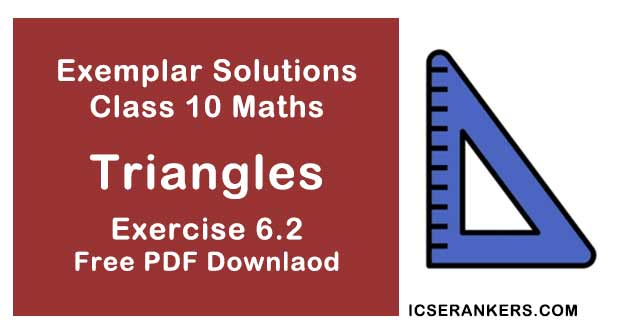Chapter Name NCERT Maths Exemplar Solutions for Chapter 6 Triangles Exercise 6.2 Book Name NCERT Exemplar for Class 10 Maths Other Exercises Exercise 6.1Exercise 6.3Exercise 6.4 Related Study NCERT Solutions for Class 10 Maths

### Exercise 6.2 Solutions

#### Short Answer Questions with Reasoning

1. Is the triangle with sides 25 cm, 5 cm and 24 cm a right triangle? Give reason for your answer.

Solution

It is not true.
Taking,
a = 25 cm,
b = 5 cm and
c = 24 cm
Now,
b2 + c2 = (5)2 + (24)2
= 25 + 576 = 601
≠ (25)2
Therefore, given sides do not make a right triangle because it does not satisfy the property of Pythagoras theorem.

2. It is given that ΔDEF ~ ΔRPQ. Is it true to say that ∠D = ∠R and ∠F = ∠P? Why?

Solution

It is not true
We know that, if two triangles are similar, then their corresponding angles are equal.
∠D = ∠R,
∠E = ∠P and
∠F = Q

3. A and B are respectively the points on the sides PQ and PR of a ΔPQR such that PQ = 12.5 cm, PA = 5 cm, BR = 6 cm and PB = 4 cm. Is AB || QR?

Solution

It is correct.
Given,
PQ = 12.5 cm,
PA = 5 cm,
BR = 6 cm and
PB = 4 cm
Also,
PB/BR = 4/6 = 2/3
So, QA = QP - PA
= 12.5 - 5 = 7.5  cm
PA/AQ = 5/7.5
= 2/3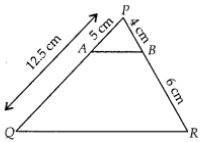So by converse of basic proportionality theorem, AB || QR.

4. In figure, BD and CE intersect each other at the point P. Is ΔPBC ~ ΔPDE? Why?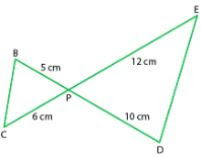Solution

It is correct.
In ΔPBC and ΔPDE,
∠BPC = ∠EPD  [vertically opposite angles]
PB/PD = 5/10 = 1/2
PC/PE = 6/12 = 1/2
So,
PB/PD = PC/PE
As, one angle of ∆PBC is equal to one angle of ∆PDE and the sides including these angles are proportional, so both triangles are similar.
So, ∆PBC ~ ∆PDE, by SAS similarity criterion.

5. In ∆PQR  and ∆MST, ∠P = 55°, ∠Q =25°, ∠M = 100° and ∠S = 25°. Is  ΔQPR ~ ΔTSM?  Why?

Solution

It is not true.
As, the sum of three angles of a triangle is 180° .
In ∆PQR,
∠P + ∠Q + ∠R = 180°
⇒ 55° + 25° + ∠R = 180°
⇒ ∠R = 180° - (55° + 25°)
⇒ ∠R = 180° - 80° = 100°

In ∆TSM,
∠T + ∠S + ∠M = 180°
⇒ ∠T + ∠25° + 100° = 180°
⇒ ∠T = 180° – (25° + 100°)
⇒ ∠T= 180° – 125°
⇒ ∠T= 55°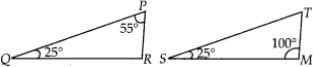So,
In ∆PQR and ∆TSM,
∠P = ∠T,
∠Q = ∠S and
∠R = ∠M
∠PQR = ∠TSM [As, all corresponding angles are equal]
Therefore,
∆QPR is not similar to ∆TSM, because correct correspondence is P ↔ T, Q ↔ S and R ↔ M.

6. Is the following statement true? Why?
“Two quadrilaterals are similar, if their corresponding angles are equal”.

Solution

It is not true.
Two quadrilaterals are similar if their corresponding angles are equal and corresponding sides must also be proportional.

7. Two sides and the perimeter of one triangle are respectively three times the corresponding sides and the perimeter of the other triangle. Are the two triangles similar? Why?

Solution

Yes, It is true.
The corresponding two sides and the perimeters of two triangles are proportional, then the third side of both triangles will also in proportion.

8. If in two right triangles, one of the acute angles of one triangle is equal to an acute angle of the other triangle, can you say that the two triangles will be similar? Why?

Solution

It is false.
Let two right angled triangles be ∆ABC and ∆PQR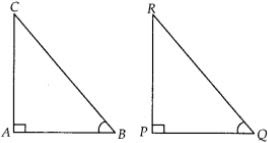Where,
∠A = ∠P = 90° and
∠B = ∠Q = acute angle (Given)
So, by AA similarity criterion, ∆ABC ~ ∆PQR

9. The ratio of the corresponding altitudes of two similar triangles is 3/5. Is it correct to say that ratio of their areas is 6/5 ? Why ?

Solution

It is false.
Ratio of corresponding altitudes of two triangles having areas A1 and A2 respectively is 3/5.  Using the property of area of two similar triangles,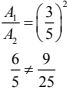So, the given statement is not correct.

10. D is a point on side QR of ∆PQR such that PD ⊥ QR. Will it be correct to say that ∆PQD ～∆RPD ? Why ?

Solution

No, it is false statement.
In given ∆PQD and ∆RPD,
PD = PD  [common side]
∠PDQ = ∠PDR [each 90°]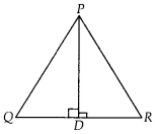Also, no other sides or angles are equal, so we can say that ∆PQD is not similar to ∆RPD.
But if ∠P = 90° , then
∠DPQ = ∠PRD
[each equal to 90° - ∠Q and by ASA similarity criterion, ∆PQD ～∆RPD]

11. In Fig. 6.5, if ∠D = ∠C, then is it true that ∆ADE ～∆ACB ? Why ?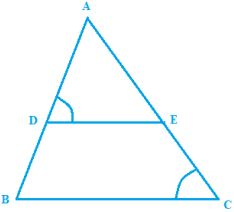Solution
True